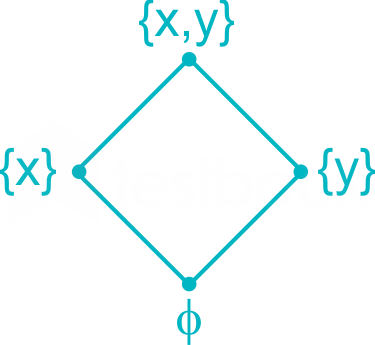266 viewsI Have doubt about the language. Is it asking about the sum of elements if we make the GBL set for the given lattice .

### 1 comment

Is it D?

I think they are asking no of element in GLB.

It should be 0

@Arkaprava

i mean to say summing the element in the set

This question is poorly framed The GBL set would comprise of {{$\varnothing$},{$x$},{$y$}}. My question is that the elements in the GBL are sets, we can't add sets. That is why I thought the answer should be 0. What is the explanation provided in the answer?

Hasse diagram:Greatest Lower Bound of lattice is { } or ϕ

Sum of elements in GLB of lattice = 0

in test book exp nothing is clear according to me x+y but it also true we not add set.

i think question is wrongly frame.

1
159 views